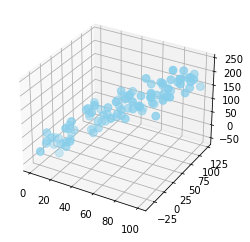In order to create a 3d graph, you should set projection parameter with '3d' keyword. Note that most of the customisations presented in the Scatterplot section will work in 3D as well. The result can be a bit disappointing since each marker is represented as a dot, not as a sphere..

``````# libraries
from mpl_toolkits.mplot3d import Axes3D
import matplotlib.pyplot as plt
import numpy as np
import pandas as pd

# Dataset
df=pd.DataFrame({'X': range(1,101), 'Y': np.random.randn(100)*15+range(1,101), 'Z': (np.random.randn(100)*15+range(1,101))*2 })

# plot
fig = plt.figure()